Next: Complex sinusoids Up: Time shifts and delays Previous: Time shifts and delays   Contents   Index

# Complex numbers

Complex numbers are written as:where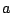andare real numbers and. (In this book we'll use the upper case Roman letters such asto denote complex numbers. Real numbers appear as lower case Roman or Greek letters, except for integer bounds, usually written asor.) Since a complex number has two real components, we use a Cartesian plane (in place of a number line) to graph it, as shown in Figure 7.1. The quantitiesandare called the real and imaginary parts of, written as: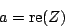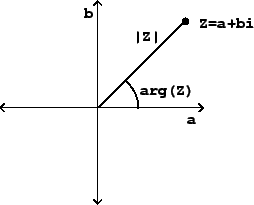Ifis a complex number, its magnitude (or absolute value), written as, is just the distance in the plane from the origin to the point:and its argument, written as, is the angle from the positiveaxis to the point:If we know the magnitude and argument of a complex number (call themand) we can reconstruct the real and imaginary parts: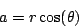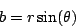A complex number may be written in terms of its real and imaginary partsand, as(this is called rectangular form), or alternatively in polar form, in terms ofand:The rectangular and polar formulations are interchangeable; the equations above show how to computeandfromandand vice versa.

The main reason we use complex numbers in electronic music is because they magically automate trigonometric calculations. We frequently have to add angles together in order to talk about the changing phase of an audio signal as time progresses (or as it is shifted in time, as in this chapter). It turns out that, if you multiply two complex numbers, the argument of the product is the sum of the arguments of the two factors. To see how this happens, we'll multiply two numbersand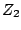, written in polar form:giving: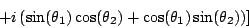Here the minus sign in front of the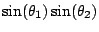term comes from multiplyingby itself, which gives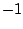. We can spot the cosine and sine summation formulas in the above expression, and so it simplifies to:By inspection, it follows that the producthas magnitudeand argument.

We can use this property of complex numbers to add and subtract angles (by multiplying and dividing complex numbers with the appropriate arguments) and then to take the cosine and sine of the result by extracting the real and imaginary parts.

SubsectionsNext: Complex sinusoids Up: Time shifts and delays Previous: Time shifts and delays   Contents   Index
Miller Puckette 2006-12-30